# Recurring Decimals (2)

In this worksheet, students convert recurring decimals to fractions.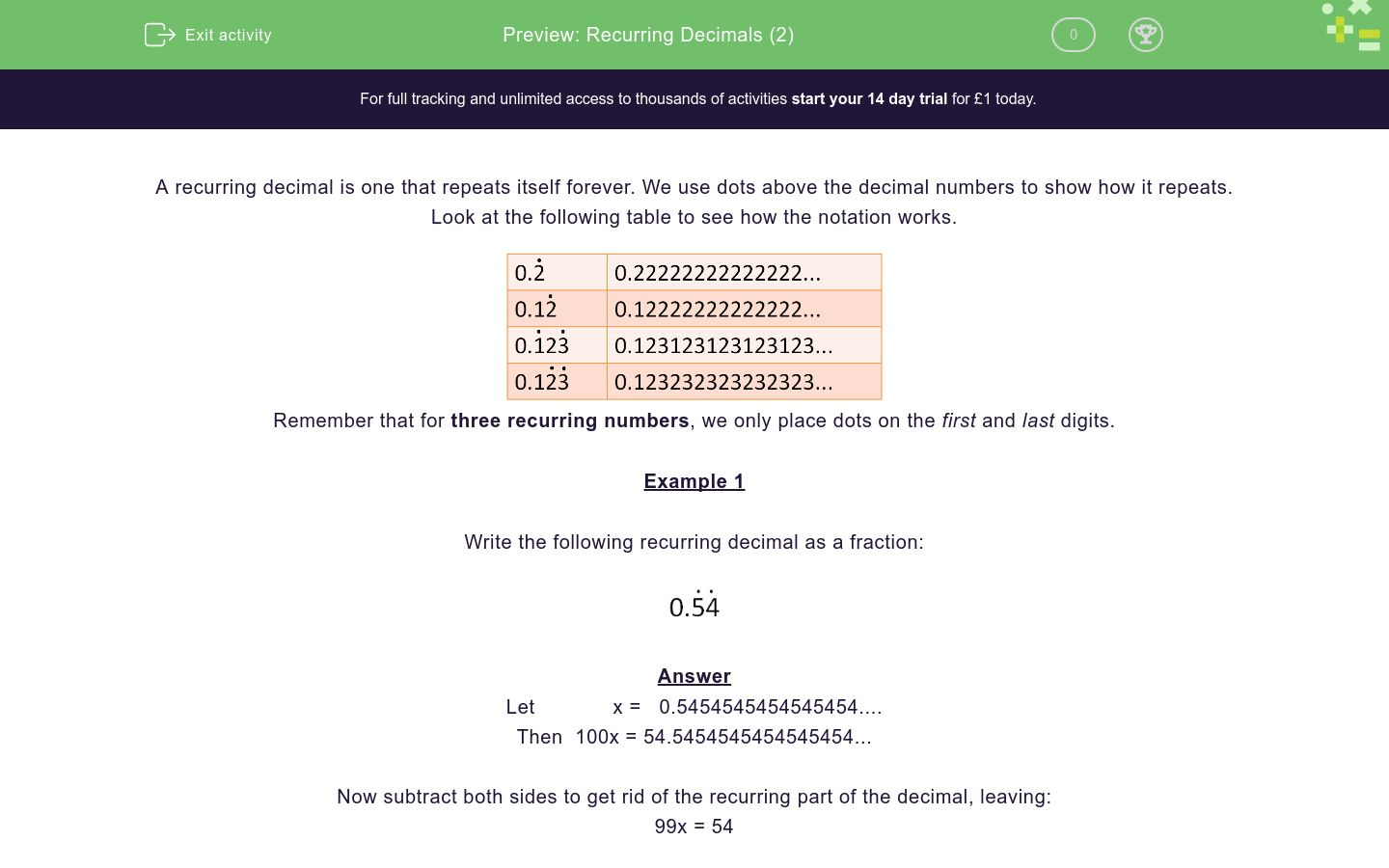Key stage:  KS 4

Curriculum topic:  Number

Curriculum subtopic:  Change Recurring Decimals into Their Corresponding Fractions and Vice Versa

Difficulty level:### QUESTION 1 of 10

A recurring decimal is one that repeats itself forever. We use dots above the decimal numbers to show how it repeats. Look at the following table to see how the notation works.Remember that for three recurring numbers, we only place dots on the first and last digits.

Example 1

Write the following recurring decimal as a fraction:Let             x =   0.5454545454545454....

Then  100x = 54.5454545454545454...

Now subtract both sides to get rid of the recurring part of the decimal, leaving:

99x = 54

So x = 54/99 = 6/11 in its lowest terms.

Example 2

Write the following recurring decimal as a fraction:Let             x =     0.405405405405....

Then  1000x = 405.405405405405...

Notice that because 3 digits recur, we have to find 1000x not 100x.

Now subtract both sides to get rid of the recurring part of the decimal, leaving:

999x = 405

So x = 405/999 = 15/37 in its lowest terms.

Notice that....=   5/9=   54/99=   405/999

Write the following recurring decimal as a fraction in its lowest terms.(Write your answer as a reduced fraction in the form a/b)

Write the following recurring decimal as a fraction in its lowest terms.(Write your answer as a reduced fraction in the form a/b)

Write the following recurring decimal as a fraction in its lowest terms.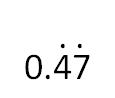(Write your answer as a reduced fraction in the form a/b)

Write the following recurring decimal as a fraction in its lowest terms.(Write your answer as a reduced fraction in the form a/b)

Write the following recurring decimal as a fraction in its lowest terms.(Write your answer as a reduced fraction in the form a/b)

Write the following recurring decimal as a fraction in its lowest terms.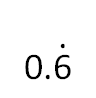(Write your answer as a reduced fraction in the form a/b)

Write the following recurring decimal as a fraction in its lowest terms.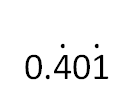(Write your answer as a reduced fraction in the form a/b)

Write the following recurring decimal as a fraction in its lowest terms.(Write your answer as a reduced fraction in the form a/b)

Write the following recurring decimal as a fraction in its lowest terms.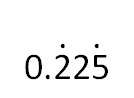(Write your answer as a reduced fraction in the form a/b)

Write the following recurring decimal as a fraction in its lowest terms.(Write your answer as a reduced fraction in the form a/b)

• Question 1

Write the following recurring decimal as a fraction in its lowest terms.(Write your answer as a reduced fraction in the form a/b)

5/9
EDDIE SAYS
One digit recurs, so 5 over 9, which doesn't reduce.
• Question 2

Write the following recurring decimal as a fraction in its lowest terms.(Write your answer as a reduced fraction in the form a/b)

8/9
EDDIE SAYS
One digit recurs, so 8 over 9, which doesn't reduce.
• Question 3

Write the following recurring decimal as a fraction in its lowest terms.(Write your answer as a reduced fraction in the form a/b)

47/99
EDDIE SAYS
Two digits recur, so 47 over 99, which doesn't reduce.
• Question 4

Write the following recurring decimal as a fraction in its lowest terms.(Write your answer as a reduced fraction in the form a/b)

5/11
EDDIE SAYS
Two digits recur, so 45 over 99, which reduces.
Divide top and bottom by 9.
• Question 5

Write the following recurring decimal as a fraction in its lowest terms.(Write your answer as a reduced fraction in the form a/b)

8/11
EDDIE SAYS
Two digits recur, so 72 over 99, which reduces.
Divide top and bottom by 9.
• Question 6

Write the following recurring decimal as a fraction in its lowest terms.(Write your answer as a reduced fraction in the form a/b)

2/3
EDDIE SAYS
One digit recurs, so 6 over 9, which reduces.
Divide top and bottom by 3.
• Question 7

Write the following recurring decimal as a fraction in its lowest terms.(Write your answer as a reduced fraction in the form a/b)

401/999
EDDIE SAYS
Three digits recur, so 401 over 999, which does not reduce as 401 is prime.
• Question 8

Write the following recurring decimal as a fraction in its lowest terms.(Write your answer as a reduced fraction in the form a/b)

15/37
EDDIE SAYS
Three digits recur, so 405 over 999, which reduces.
Divide top and bottom by 27 or by 9 and 3 in stages.
• Question 9

Write the following recurring decimal as a fraction in its lowest terms.(Write your answer as a reduced fraction in the form a/b)

25/111
EDDIE SAYS
Three digits recur, so 225 over 999, which reduces.
Divide top and bottom by 9.
• Question 10

Write the following recurring decimal as a fraction in its lowest terms.(Write your answer as a reduced fraction in the form a/b)

1/7
EDDIE SAYS
Six digits recur, so 142857 over 999999, which reduces.
Divide top and bottom by 9 to get 15873/111111.
Divide top and bottom by 11 to get 1443/10101.
Divide top and bottom by 13 to get 111/777.
Divide top and bottom by 111 to get 1/7.
Or just remember the recurring decimal for one seventh!
---- OR ----

Sign up for a £1 trial so you can track and measure your child's progress on this activity.

### What is EdPlace?

We're your National Curriculum aligned online education content provider helping each child succeed in English, maths and science from year 1 to GCSE. With an EdPlace account you’ll be able to track and measure progress, helping each child achieve their best. We build confidence and attainment by personalising each child’s learning at a level that suits them.

Start your £1 trial